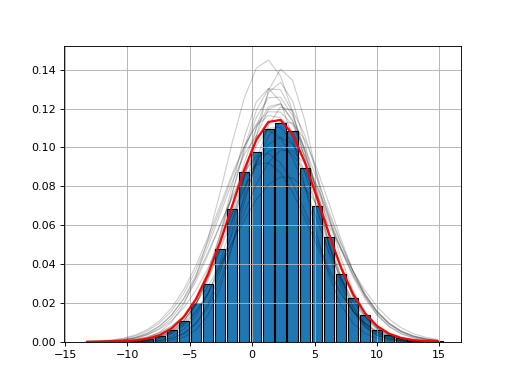# Examples¶

Let us start with an example. We generate a vector of values from a gamma distribution.

```from scipy import stats
data = stats.gamma.rvs(2, loc=1.5, scale=2, size=100000)

from fitter import Fitter
f = Fitter(data, distributions=['gamma', 'rayleigh', 'uniform'])
f.fit()
f.summary()
```

Here, we restrict the analysis to only 3 distributions by providing the list of distributions to consider. If you do not provide that parameter, 80 distributions will be considered (the analysis will be longer) and computation make take a while to finish.

The `fitter.fitter.Fitter.summary()` method shows the first best distributions (in terms of fitting).

Once the fitting is performed, one may want to get the parameters corresponding to the best distribution. The parameters are stored in `fitted_param`. For instance in the example above, the summary told us that the Gamma distribution has the best fit. You would retrieve the parameters of the Gamma distribution as follows:

```>>> f.fitted_param['gamma']
(1.9870244799532322, 1.5026555566189543, 2.0174462493492964)
```

Here, you will need to look at scipy documentation to figure out what are those parameters (mean, sigma, shape, …). For convenience, we do provide the corresponding PDF:

```f.fitted_pdf['gamma']
```

but you may want to plot the gamma distribution yourself. In that case, you will need to use Scipy package itself. Here is an example

```from pylab import linspace, plot
import scipy.stats

dist = scipy.stats.gamma
param = (1.9870, 1.5026, 2.0174)
X = linspace(0,10, 10)
pdf_fitted = dist.pdf(X, *param)
plot(X, pdf_fitted, 'o-')
```

## HistFit class: fit the density function itself¶

Sometimes, you only have the distribution itself. For instance:

```import scipy.stats
data = [scipy.stats.norm.rvs(2,3.4) for x in  range(10000)]
Y, X, _ = hist(data, bins=30)
```